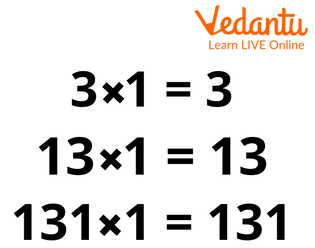Courses
Courses for Kids
Free study material
Free LIVE classes
More

# Every Number is a Factor of ItselfLIVE
Join Vedantu’s FREE Mastercalss

## Introduction

We know about factors, a number that divides other numbers without leaving any reminder called a factor. There are many properties related to the factors; one of these properties is that every number is a factor of itself. So now let us discuss what this property means.Showing Factors of 18

## How Every Number is a Factor of Itself:

Factors are those numbers that divide a number completely, so a number can divide itself completely, hence every number is a factor of itself. So let’s discuss this property with the help of an example. Let’s take example of some numbers in which we have to find the factors of that numbers, let’s say those number are 1,2,3,5,7,9,12,25

Factors of 1–1

Factors of 2–1,2

Factors of 3–1,3

Factors of 4–1,2,4

Factors of 5–1,5

Factors of 7–1,7

Factors of 9–1,3,9

Factors of 12–1, 2,3,4,6,12

Factors of 25–1, 5,25

Hence,from these examples we can conclude that 1 Is a factor of every number and every number is a factor of itself.Showing Every Number is a Factor of Itself

## Why Integers have Finite Number of Factors:

A number can be said as a factor of another number if it can be expressed as a product of that number. We know that the factors of a number is always less than or equal to the number.Let’s understand this with the help of example:

Factors of 40 –1,2,4,5,8,10,20,40

So from this example we can conclude that factors of another number can lie between one and the number itself, As every number is a finite number so factors of that number which lie between 1 and the number itself are finite.

## Why Factor of Number is Always Less than or Equal to the Number:

Factor of a number is nothing but just a part of the number as any part of a number cannot be greater than the number itself. That's why the factor of a number is always less than or equal to the number. Let’s understand this with the help of an example: let I have a pizza now cut the pizza into slices, any slice of the pizza cannot be greater than the pizza itself, same is the case of factors as any factor a number cannot be greater than the number.

Factors of 8–1,2,4,8

Factors of 16–1,2,4,8,16

From the above examples it is clear that the factor of a number cannot be greater than the number itself; they are always less than or equal to the number.Showing Factors of Every Number is always Less Than or Equal to the Number

## Exceptional Numbers which do not have at least Two Factors:

We know that the factor of every number is always 1 and the number itself in case of prime numbers, in case of numbers that are other than prime number factors may be more than two.0 and 1 are two exceptional numbers which do not have at least two factors. We know that the smallest prime number is 2 so in this case zero and one or not prime numbers because prime numbers are those numbers which are divided by 1 and the number itself. In case of 1 the factor is only 1 itself. 0 too is not a prime number.

## Conclusion:

In this article we learned about some properties of factors along with some examples which prove the properties. In this we learned that every prime number has at least two factors and composite numbers can have more than two factors which cannot be greater than the number itself. An exceptional number which does not have at least two factors is 0 and 1.

Last updated date: 27th Sep 2023
Total views: 70.5k
Views today: 0.70k

## FAQs on Every Number is a Factor of Itself

1. Numbers which do not have at least two factors?

0 and 1 are two numbers which do not have at least two factors.

2. Methods of finding factors of numbers?

Factors of a number can be found using division method and multiplication method.

3.What are prime factors?

Prime numbers which are factors of a number are called prime factors.

Example – prime factors of 15 –3,5

4. Why are factors always whole numbers?

Factors of a number cannot be decimal because factors are those numbers which divide another number without leaving any reminder.

5. Find the common factor of 5 and 8?

Factors of 5 – 1,5

Factors of 8–1,2,4,8

Common factor of 5 and 8 is 1.